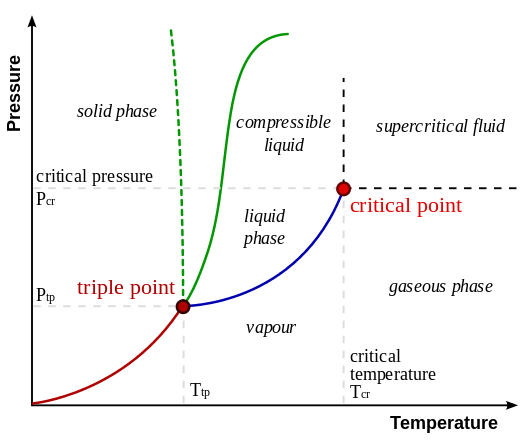# Difference Between Critical Point and Triple Point

## Main Difference – Critical Point vs Triple Point

The phase of a substance is determined by pressure and temperature of that substance. Therefore, the phase of a given substance can be changed by changing either the temperature or pressure or both. The critical point and the triple point of a substance are two important combinations of temperature and pressure. The critical point of a substance lies at the endpoint of the phase equilibrium curve whereas the triple point is the point where the three equilibrium curves meet. At the critical point of a substance, only gaseous and liquid phases can exist in equilibrium. But at the triple point, all three phases of the substance can exist. This is the main difference between critical point and triple point.

1. What is Critical Point? – Definition and Explanation

2. What is Triple Point? – Definition  and Explanation

3. What is the difference between Critical Point and Triple Point? – Comparison of Key Features## What is Critical Point

The critical point of a given substance occurs at the endpoint of its phase equilibrium curve. The critical point of a substance is defined by its critical temperature and the critical pressure. In order to understand what is meant by “the critical point” exactly, one should have a clear idea about phase diagrams.

A phase diagram is simply a graph of pressure versus temperature, which represents the behavior of a given substance under different combinations of pressure and temperature. There are three important curves on a phase diagram which are known as fusion line, sublimation line, and the vaporization line.

Fusion line is the line along which the solid and liquid phases of a given substance can exist in equilibrium. The solid and gaseous phases can exist in equilibrium along the sublimation line. Liquid and gaseous phases can exist in equilibrium along the vaporization line.

The top limit of the liquid- vapor equilibrium curve or the vaporization line is called the “critical point”.  The temperature and pressure corresponding to the critical point of a substance are known as the critical temperature and the critical pressure respectively.

The critical point of a substance is a special point on its phase diagram. It is a unique point for a given substance and differs from substance to substance. If someone increased the pressure on a vapor (gas) keeping the sample at a temperature between the critical temperature and the temperature corresponding to the triple point, the substance would cross the vaporization line and eventually would condense into a liquid.

Above the critical temperature and pressure, the sample is in a supercritical state, which is a highly pressurized gas. In other words, it is impossible to condense a gas into a liquid just by increasing the pressure, if the temperature is higher than the critical temperature.O-A Represents the fusion line

A-C Represents the vaporization line

O-A Represents the sublimation line

A-Triple point

C-Critical point

## What is Triple Point

In thermodynamics, the triple point of a given substance is an important point that lies on the phase diagram of that substance. This special point can be defined as the pressure and temperature at which the three phases of a given substance (solid, liquid and gaseous) coexist in equilibrium.

The triple point of a substance can be easily identified. It is the point at which all three lines meet. Therefore, at the triple point of a substance, all three phases exist in equilibrium.

At the triple point, six processes take place at the same time. They are evaporation, condensation, fusion, freezing, sublimation, and deposition. The equilibrium of the six processes vanishes as the temperature or pressure (or both) of the system changes.

The triple point differs from substance to substance. For example, the triple point of water occurs at 0.010 C (273.16 K) and 611.22 Pa. But it is not the triple point of Iron, Copper or Lead.The solid green line shows the usual shape of the liquid-solid phase line. The dotted green line shows the behavior of water.

## Difference Between Critical Point and Triple Point

### Definition

Critical point: The critical point is the endpoint of its phase equilibrium curve and defined by its critical temperature and the critical pressure.

Triple point: The triple point can be defined as the pressure and temperature at which the three phases of a given substance coexist in equilibrium.

### Relationship with Standard Temperature

Critical point: The critical temperature is usually higher than the standard temperature. (True for most of the substances)

Triple point: The temperature corresponding to the triple point is usually lower than the standard temperature. (True for most of the substances).

### Relationship with Standard Pressure

Critical point: Critical pressure is generally higher than the standard pressure.

Triple point: The pressure corresponding to the triple point is  generally lower than the standard pressure.

### Physical Significance

Critical point: At the critical point, only liquid and gaseous phases can coexist in equilibrium.

Triple point: All solid, liquid, and gaseous phases can coexist in equilibrium.

Image Courtesy:

“Phase-diag2” By Matthieumarechal (CC BY-SA 3.0) via Commons Wikimedia